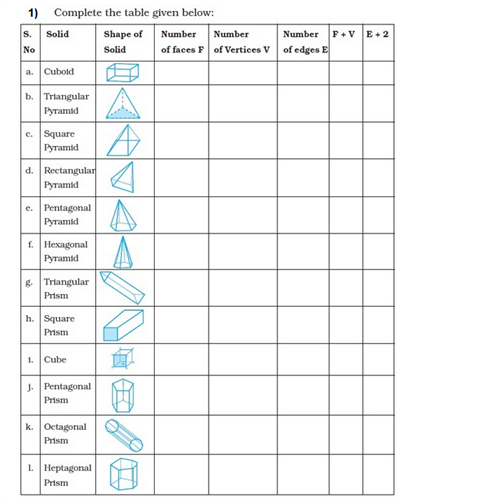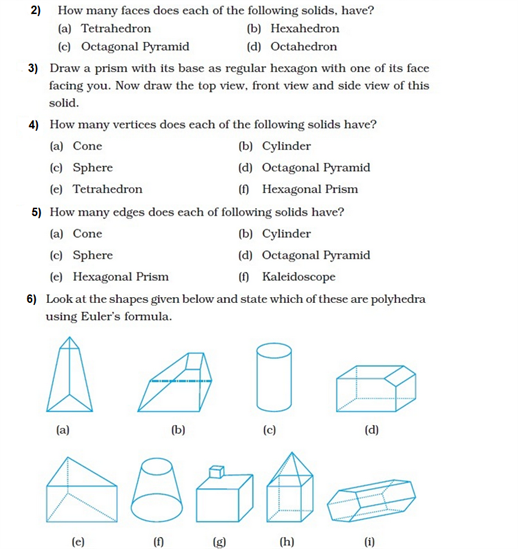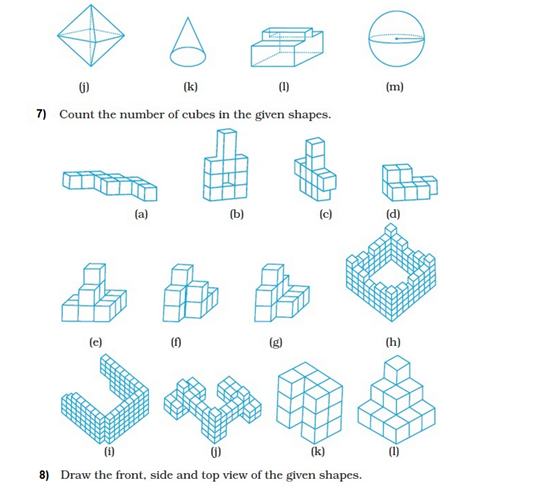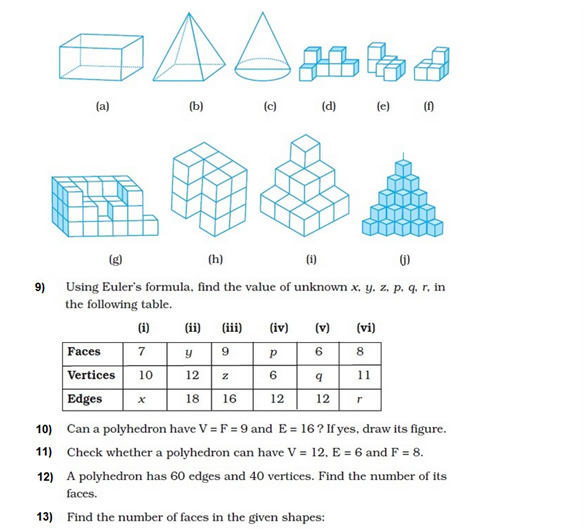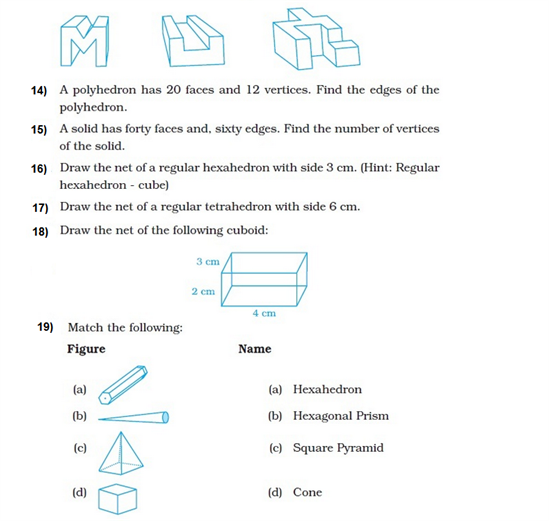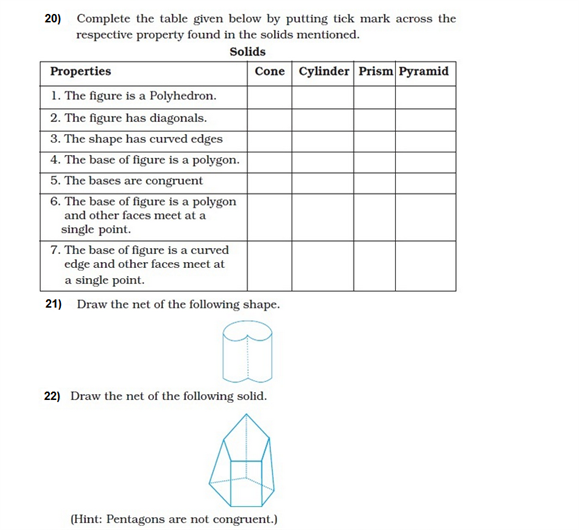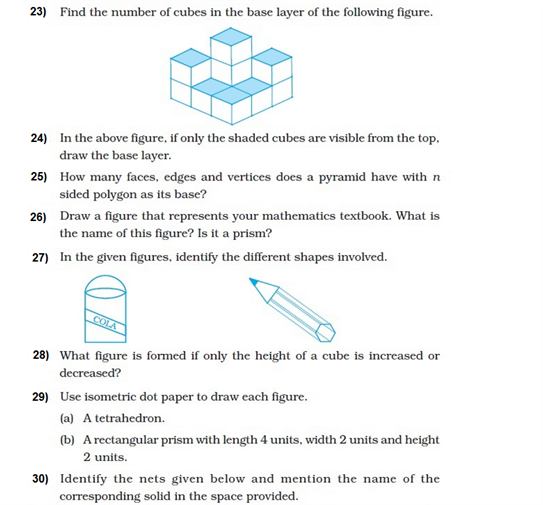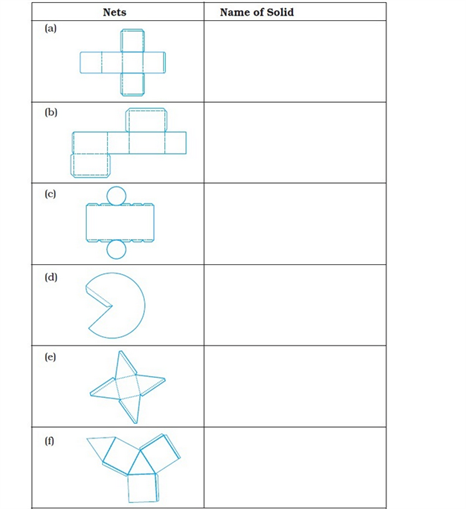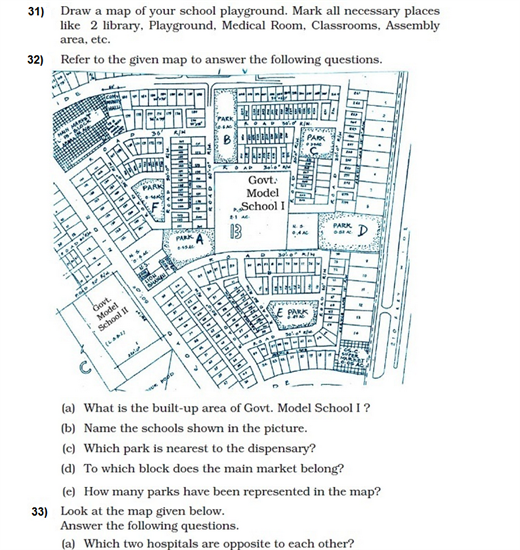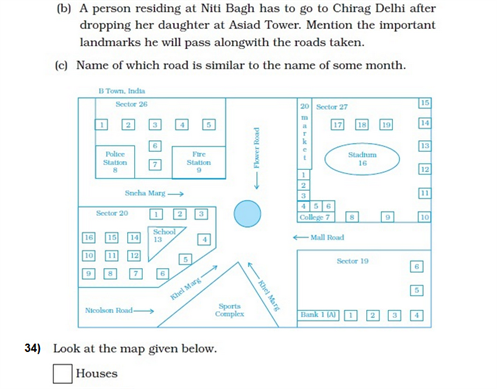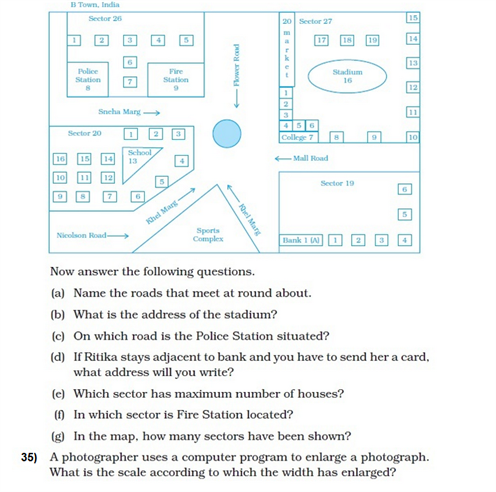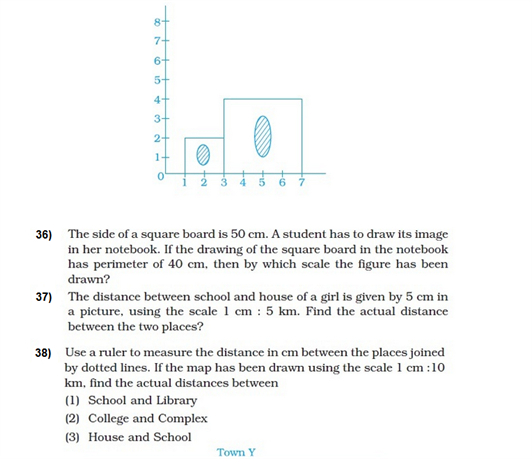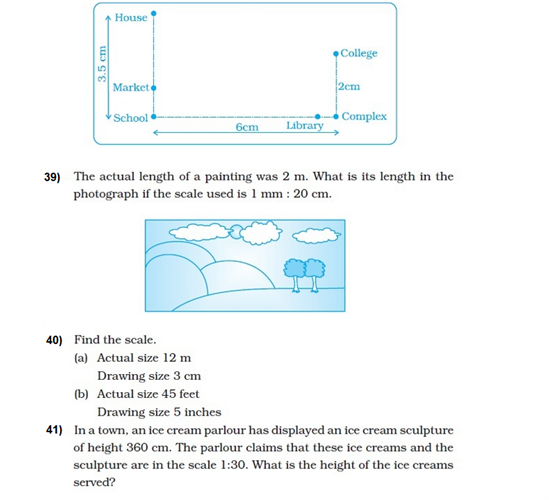# Important Questions Class 8 Maths Chapter 10 Visualising Solid Shapes

Some important class 8 maths questions from visualizing solid shapes i.e. chapter 10 are provided here to help the students prepare better for the class 8 CBSE exams. These questions require the students to be thorough with the concepts of three dimensional shapes and will eventually help them to develop skills in solving related questions more effectively.

Also Check:

## Important Visualizing Solid Shapes Questions for Class 8 Chapter 10 (With Solutions)

1. How many faces, edges and vertices do a triangular prism and a triangular pyramid has?

Solution:

The number of faces, edges and vertices of a triangular prism are:

 Shape Faces Edges Vertices Triangular Prism 6 12 8 Triangular Pyramid 4 6 4

2. What is Euler’s formula for polyhedra?

Solution:

Euler’s formula for polyhedra states that for nay solid shape, number of vertices (V) minus the number of edges (E) plus the number of faces (F) always equals to 2.

So, V – E + F = 2

3. A tetrahedron has 4 vertices and 6 edges. Find the number of faces it has.

Solution:

Using Euler’s formula for polyhedra,

V – E + F = 2

So, 4 – 6 + F = 2

Or, F = 4.

4. What is the minimum number of planes that are required to form a solid?

Solution:

At least 4 planes are required to form a solid. The solid formed with only 4 planes is called a tetrahedron or a triangular pyramid.

5. Find the missing numbers:

 Faces Vertices Edges 6 8 ? ? 10 15 4 ? 6 5 6 ? 8 12 ? 7 7 ?

Solution:

Use Euler’s formula to find the missing numbers: V – E + F = 2

 Faces Vertices Edges 6 8 12 7 10 15 4 4 6 5 6 9 8 12 18 7 7 12

### Extra Questions For Class 8 Chapter 10: Visualising Solid Shapes (NCERT)